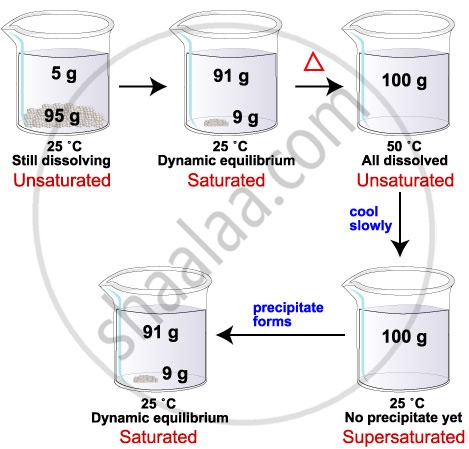# Concentration of a Solution

• Concentration of a Solution
1. Mass percentage
2. Mole fraction
3. Molarity
4. Molality
5. Volume percentage

## Definition

• Unsaturated solution: If the amount of solute contained in a solution is less than the saturation level, it is called an unsaturated solution. (till it is dissolving).

• Saturated solution: When no more solute can be dissolved in a solution at a given temperature, it is called a saturated solution.

• Solubility: The amount of the solute present in the saturated solution at this temperature is called its solubility.

## Notes

### Concentration of a Solution:• Take salt and dissolve it in water.

• After a time, it won’t be soluble.

• Heat it and try dissolving.

• It will dissolve!

• Try adding more and dissolve it.

From the about activity, the following can be inferred!

1. Unsaturated solution: If the amount of solute contained in a solution is less than the saturation level, it is called an unsaturated solution. (till it is dissolving).

2. Saturated solution: When no more solute can be dissolved in a solution at a given temperature, it is called a saturated solution.

3. Solubility: The amount of the solute present in the saturated solution at this temperature is called its solubility.

#### How will you decide how concentrated the solution is?

Concentration of solution = " Amount of solute"/"Amount of solution"  "or" "Amount of solute"/"Amount of solvent"

There are various ways of expressing the concentration of a solution

1. "Mass by mass percentage of a solution" = "Mass of solute"/"Mass of solution" xx 100

2. "Mass by volume percentage of a solution" = "Mass of solute"/"Volume of solution" xx 100.

## Example

A solution contains 40 g of common salt in 320 g of water. Calculate the concentration in terms of mass by the mass percentage of the solution.
Mass of solute (salt) = 40 g
Mass of solvent (water) = 320 g
We know,
Mass of solution = Mass of solute + Mass of solvent
= 40 g + 320 g
= 360 g
Mass percentage of solution = "Mass of solute"/"Mass of solution" xx 100
= 40/360 xx 100
= 11.1%

## Example

To make a saturated solution, 36 g of sodium chloride is dissolved in 100 g of water at 293 K. Find its concentration at this temperature.

Mass of solute (sodium chloride) = 36 g (Given)

Mass of solvent (water) = 100 g (Given)

Then, mass of solution = Mass of solute + Mass of solvent

= (36 + 100) g

= 136 g

Therefore, concentration (mass by mass percentage) of the solution

="Mmmmass of solute"/"Mass of solvent"xx100%

=36/136xx100%

= 26.47 %

If you would like to contribute notes or other learning material, please submit them using the button below.

### Shaalaa.com

Solubility of Solution [00:11:32]
S
0%